Do you like the article?
Share it with others -

Use new possibilities of MetaTrader 5

# PairPlot graph based on CGraphic for analyzing correlations between data arrays (time series)

25 September 2018, 14:46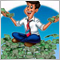0
16 367

### Introduction

As we all know, forex traders perform transactions using currency pair quotes, in which the currency value of one country is expressed in the currency of another. It is easy to see that the same currency can be found in several currency pairs. Since the value of a currency is determined by the economic state of its country, we may ask whether changes in the country's economy affect its currency uniformly in different currency pairs. A positive answer seems inevitable here. But it is justified only in a perfect case when the economic state of only one country changes. The truth of life is that our world is constantly changing. A change in the economy of one country directly or indirectly entails a change in the global economy.

On the Internet, you can find plenty of information about analyzing changes of a currency price within various pairs and searching for correlation between different currency pairs. On this website, you can find articles about trading currency pair baskets [1, 2, 3]. Nevertheless, the question of analyzing the correlations between time series remains open. In this article, I suggest developing a tool for graphical analysis of correlations between time series, which allows visualizing correlations between time series of quotes of analyzed currency pairs.

Before we get to work, let's define our objectives. What kind of tool do we want to obtain in the end? First of all, it should be a graphical panel containing graphs of correlations between passed time series. The tool should be versatile enough and able to work with different number of time series.

To analyze the time series on the panel, we will build a distribution histogram for each time series. We will also prepare scatter plots to be displayed in pairs for analyzed time series in order to search for a correlation. Trend lines will be added to scatter plots as a visual reference.

The layout of the graphs in the form of a cross table will improve the readability of the entire tool. This approach unifies the data presentation and simplifies visual perception. The layout of the proposed tool is provided below.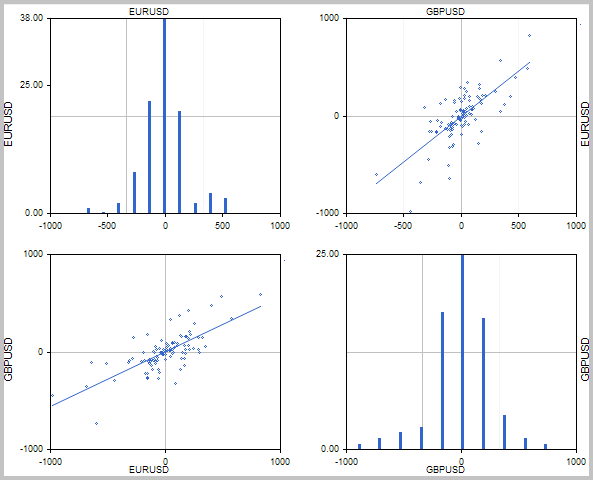### 2. Creating base classes

#### 2.1. "The basis"

While developing such a tool, we should keep in mind that users may work with a different number of trading instruments. I believe, the perfect visual solution is a block-based representation where standard graphs are used as "bricks" for building a common correlation table.

We will start developing our tool by preparing the basis for charts construction. MetaTrader 5 basic delivery features the CGraphic class meant for building scientific graphs. The article  provides a detailed description of this class. We will use it as the basis for constructing our graphs. Let's create the CPlotBase base class and assign it as the one derived from the CGraphic standard class. In this class, we will create methods for creating a graph canvas. There will be two such methods: the first one is to plot a square graph field with a given side dimension and the second one is to construct a rectangular area using given coordinates. We will also add the methods for displaying a text on the sides of the graph (they will help us in displaying the names of instruments). Besides, let's add the method for changing the display color on the time series graph.

We should also keep in mind that the applied CGraphic base class is not derived from the CObject class and does not contain the methods for relocating, hiding and displaying an object on a graph. Similar methods are widely used in graphical panels. Therefore, we need to add these methods to the created class for the compatibility of our tool with the standard classes for building graphical panels.

```class CPlotBase : public CGraphic
{
protected:
long              m_chart_id;                // chart ID
int               m_subwin;                  // chart subwindow

public:
CPlotBase();
~CPlotBase();
//--- Create of object
virtual bool      Create(const long chart,const string name,const int subwin,const int x1,const int y1,const int size);
virtual bool      Create(const long chart,const string name,const int subwin,const int x1,const int y1,const int x2,const int y2);
//--- Change color of timeserie's label
virtual bool      SetTimeseriesColor(uint clr, uint timeserie=0);
virtual void      TextUp(string text, uint clr);
virtual void      TextDown(string text, uint clr);
virtual void      TextLeft(string text, uint clr);
virtual void      TextRight(string text, uint clr);
//--- geometry
virtual bool      Shift(const int dx,const int dy);
//--- state
virtual bool      Show(void);
virtual bool      Hide(void);
};```

In the class constructor, remove the graph legend display and set the minimum number of labels along the axes.

```CPlotBase::CPlotBase()
{
HistoryNameWidth(0);
HistorySymbolSize(0);
m_x.MaxLabels(3);
m_y.MaxLabels(3);
}```

Find the entire code of all class methods in the attachment.

#### 2.2. Scatter plot

Next, develop the CScatter class for displaying the scatter plot. This class will only contain two methods for creating and updating the time series data.

```class CScatter : public CPlotBase
{

public:
CScatter();
~CScatter();
//---
int               AddTimeseries(const double &timeseries_1[],const double &timeseries_2[]);
bool              UpdateTimeseries(const double &timeseries_1[],const double &timeseries_2[],uint timeserie=0);

};```

Two time series arrays of analyzed instruments the scatter plot is based on are to be passed to the AddTimeseries curve creation method. The CGraphic standard class is able to display a point graph based on two data arrays. We will use this feature. At the beginning of the method, create a point curve based on two data arrays. If the curve creation fails, exit the function with the result "-1". If the curve is successfully created, set the size of the curve points and set the trend line display flag. After performing all operations, the method returns the index of the created curve.

```int CScatter::AddTimeseries(const double &timeseries_1[],const double &timeseries_2[])
{
if(curve==NULL)
return -1;
curve.PointsSize(2);
curve.TrendLineVisible(true);
return (m_arr_curves.Total()-1);
}
```

To update the curve data, create the UpdateTimeseries method. Two data arrays for creating the curve and the index of the curve, the data of which should be changed, are to be passed to it. At the beginning of the function, check the validity of the specified curve number. If the number is specified erroneously, exit the function with 'false'.

Then, compare the dimension of the received time series. If array sizes are different or the arrays are empty, end the function with 'false'.

The next step is specifying the pointer to a curve object by index. If the pointer is incorrect, end the function with 'false'.

After all the checks, pass the time series to the curve and end the function with 'true'.

```bool CScatter::UpdateTimeseries(const double &timeseries_1[],const double &timeseries_2[], uint timeserie=0)
{
if((int)timeserie>=m_arr_curves.Total())
return false;
if(ArraySize(timeseries_1)!=ArraySize(timeseries_2) || ArraySize(timeseries_1)==0)
return false;
//---
CCurve *curve=m_arr_curves.At(timeserie);
if(CheckPointer(curve)==POINTER_INVALID)
return false;
//---
curve.Update(timeseries_1,timeseries_2);
//---
return true;
}```

#### 2.3. Histogram

The histogram is yet another "brick" for building our tool. We need the CHistogram class to create it. Like CScatter, the class receives its own curve data generation and update methods. However, unlike its predecessor, the current class applies one time series to build the curve. The principles of constructing these methods are similar to the methods of the previous class.

Keep in mind that the CGraphic base class can build the histogram only in its standard form. To add the possibility of constructing a vertical histogram of the Market Profile type, we will have to rewrite the HistogramPlot method. Besides, we should add the e_orientation variable for storing the histogram construction type and re-write the graph canvas creation methods by adding the ability to specify the histogram type to them.

Another difference between our class and the base class in CGpraphic is the type of initial data we get. In the base class, an array of obtained values ​​is used for direct output to the graph. Our class will receive a time series, and before processing the histogram, it will be necessary to process the obtained data. Preparing the data for constructing the histogram is performed by the CalculateHistogramArray method, number of histogram columns is set by the SetCells method and saved in the i_cells variable.

```class CHistogram : public CPlotBase
{
private:
ENUM_HISTOGRAM_ORIENTATION    e_orientation;
uint                          i_cells;

public:
CHistogram();
~CHistogram();
//---
bool              Create(const long chart,const string name,const int subwin,const int x1,const int y1,const int size, ENUM_HISTOGRAM_ORIENTATION orientation=HISTOGRAM_HORIZONTAL);
bool              Create(const long chart,const string name,const int subwin,const int x1,const int y1,const int x2,const int y2, ENUM_HISTOGRAM_ORIENTATION orientation=HISTOGRAM_HORIZONTAL);
bool              UpdateTimeserie(const double &timeserie[],uint timeserie=0);
bool              SetCells(uint value)    {  i_cells=value; }

protected:
virtual void      HistogramPlot(CCurve *curve);
bool              CalculateHistogramArray(const double &data[],double &intervals[],double &frequency[],
double &maxv,double &minv);
};
```

The CalculateHistogramArray method is based on the algorithm offered in the MQL5 Reference with a small addition. At the beginning of the method, check the sufficiency of the initial data for plotting the histogram, determine the minimum and maximum values, calculate the range width of each interval and prepare arrays to store intervals and frequencies.

After that, the interval centers are set in the loop and the frequency array is set to zero.

In the next loop, iterate through the time series and count the values fitting the corresponding interval.

Finally, normalize the frequencies converting the mentioned fittings into percentage of the total number of elements in the time series.

```bool CHistogram::CalculateHistogramArray(const double &data[],double &intervals[],double &frequency[],
double &maxv,double &minv)
{
int size=ArraySize(data);
if(size<(int)i_cells*10) return (false);
minv=data[ArrayMinimum(data)];
maxv=data[ArrayMaximum(data)];
double range=maxv-minv;
double width=range/i_cells;
if(width==0) return false;
ArrayResize(intervals,i_cells);
ArrayResize(frequency,i_cells);
//--- set the interval centers
for(uint i=0; i<i_cells; i++)
{
intervals[i]=minv+(i+0.5)*width;
frequency[i]=0;
}
//--- fill in the interval fitting frequencies
for(int i=0; i<size; i++)
{
uint ind=int((data[i]-minv)/width);
if(ind>=i_cells) ind=i_cells-1;
frequency[ind]++;
}
//--- normalize frequencies into percentage
for(uint i=0; i<i_cells; i++)
frequency[i]*=(100.0/(double)size);
return (true);
} ```

The histogram is plotted on the graph by the HistogramPlot method. This function is built based on the CGraphic base class algorithm corrected for the use of time series and the histogram construction orientation.

At the beginning of the method, prepare the data for plotting the histogram. To do this, we get a time series from the curve data and call the CalculateHistogramArray method. After the function is executed successfully, we get the histogram blocks width and check the construction data array size.

Next, format the values by axes according to the histogram display type.

Finally, arrange the loop for displaying the diagram columns on the graph field.

```CHistogram::HistogramPlot(CCurve *curve)
{
double data[],intervals[],frequency[];
double max_value, min_value;
curve.GetY(data);
if(!CalculateHistogramArray(data,intervals,frequency,max_value,min_value))
return;
//--- historgram parameters
int histogram_width=fmax(curve.HistogramWidth(),2);
//--- check
if(ArraySize(frequency)==0 || ArraySize(intervals)==0)
return;
//---
switch(e_orientation)
{
case HISTOGRAM_HORIZONTAL:
m_y.AutoScale(false);
m_x.Min(intervals[ArrayMinimum(intervals)]);
m_x.Max(intervals[ArrayMaximum(intervals)]);
m_x.MaxLabels(3);
m_x.ValuesFormat("%.0f");
m_y.Min(0);
m_y.Max(frequency[ArrayMaximum(frequency)]);
m_y.ValuesFormat("%.2f");
break;
case HISTOGRAM_VERTICAL:
m_x.AutoScale(false);
m_y.Min(intervals[ArrayMinimum(intervals)]);
m_y.Max(intervals[ArrayMaximum(intervals)]);
m_y.MaxLabels(3);
m_y.ValuesFormat("%.0f");
m_x.Min(0);
m_x.Max(frequency[ArrayMaximum(frequency)]);
m_x.ValuesFormat("%.2f");
break;
}
//---
CalculateXAxis();
CalculateYAxis();
//--- calculate original of y
int originalY=m_height-m_down;
int originalX=m_width-m_right;
int yc0=ScaleY(0.0);
int xc0=ScaleX(0.0);
//--- gets curve color
uint clr=curve.Color();
//--- draw
for(uint i=0; i<i_cells; i++)
{
//--- check coordinates
if(!MathIsValidNumber(frequency[i]) || !MathIsValidNumber(intervals[i]))
continue;
if(e_orientation==HISTOGRAM_HORIZONTAL)
{
int xc=ScaleX(intervals[i]);
int yc=ScaleY(frequency[i]);
int xc1 = xc - histogram_width/2;
int xc2 = xc + histogram_width/2;
int yc1 = yc;
int yc2 = (originalY>yc0 && yc0>0) ? yc0 : originalY;
//---
if(yc1>yc2)
yc2++;
else
yc2--;
//---
m_canvas.FillRectangle(xc1,yc1,xc2,yc2,clr);
}
else
{
int yc=ScaleY(intervals[i]);
int xc=ScaleX(frequency[i]);
int yc1 = yc - histogram_width/2;
int yc2 = yc + histogram_width/2;
int xc1 = xc;
int xc2 = (originalX>xc0 && xc0>0) ? xc0 : originalX;
//---
if(xc1>xc2)
xc2++;
else
xc2--;
//---
m_canvas.FillRectangle(xc1,yc1,xc2,yc2,clr);
}
}
//---
}```

The full code of all classes and methods is available in the attachment.

#### 2.4. Class for working with time series

To build the tool, we need yet another "brick" that will download required historical data and prepare timeseries for plotting graphs. This work will be performed in the CTimeserie class. The instrument name, the timeframe and the applied price are to be passed when initializing the class. Besides, the methods for subsequent change of the instrument name, timeframe, applied price and history depth are to be created.

```class CTimeserie :  public CObject
{
protected:
string               s_symbol;
ENUM_TIMEFRAMES      e_timeframe;
ENUM_APPLIED_PRICE   e_price;
double               d_timeserie[];
int                  i_bars;

public:
CTimeserie(void);
~CTimeserie(void);
bool              Create(const string symbol=NULL, const ENUM_TIMEFRAMES timeframe=PERIOD_CURRENT, const ENUM_APPLIED_PRICE price=PRICE_CLOSE);
//--- Change settings of time series
void              SetBars(const int value)            {  i_bars=value;  }
void              Symbol(string value)                {  s_symbol=value;      dt_last_load=0;  }
void              Timeframe(ENUM_TIMEFRAMES value)    {  e_timeframe=value;   dt_last_load=0;  }
void              Price(ENUM_APPLIED_PRICE value)     {  e_price=value;       dt_last_load=0;  }
//---
string            Symbol(void)                        {  return s_symbol;     }
ENUM_TIMEFRAMES   Timeframe(void)                     {  return e_timeframe;  }
ENUM_APPLIED_PRICE Price(void)                        {  return e_price;      }
virtual bool      UpdateTimeserie(void);
bool              GetTimeserie(double &timeserie[])   {  return ArrayCopy(timeserie,d_timeserie)>0;   }
};```

The main data preparation work is to be done in the UpdateTimeserie method. At the beginning of the method, check whether the necessary information has been previously loaded on the current bar. If the information is ready, exit the function with 'true'. If it is necessary to prepare the data, upload the necessary history data in accordance with the specified price. If it is impossible to upload the information, exit the function with 'false'. Note that we are not interested in the price itself but in its change. Therefore, the history data upload exceeds the specified amount by 1 bar. At the next stage, we recalculate the price change on each bar and save it to the array in a loop. Later, a user can get this information using the GetTimeserie method.

```bool CTimeserie::UpdateTimeserie(void)
{
datetime cur_date=(datetime)SeriesInfoInteger(s_symbol,e_timeframe,SERIES_LASTBAR_DATE);
return true;
//---
MqlRates rates[];
int bars=0,i;
double data[];
switch(e_price)
{
case PRICE_CLOSE:
bars=CopyClose(s_symbol,e_timeframe,1,i_bars+1,data);
break;
case PRICE_OPEN:
bars=CopyOpen(s_symbol,e_timeframe,1,i_bars+1,data);
case PRICE_HIGH:
bars=CopyHigh(s_symbol,e_timeframe,1,i_bars+1,data);
case PRICE_LOW:
bars=CopyLow(s_symbol,e_timeframe,1,i_bars+1,data);
case PRICE_MEDIAN:
bars=CopyRates(s_symbol,e_timeframe,1,i_bars+1,rates);
bars=ArrayResize(data,bars);
for(i=0;i<bars;i++)
data[i]=(rates[i].high+rates[i].low)/2;
break;
case PRICE_TYPICAL:
bars=CopyRates(s_symbol,e_timeframe,1,i_bars+1,rates);
bars=ArrayResize(data,bars);
for(i=0;i<bars;i++)
data[i]=(rates[i].high+rates[i].low+rates[i].close)/3;
break;
case PRICE_WEIGHTED:
bars=CopyRates(s_symbol,e_timeframe,1,i_bars+1,rates);
bars=ArrayResize(data,bars);
for(i=0;i<bars;i++)
data[i]=(rates[i].high+rates[i].low+2*rates[i].close)/4;
break;
}
//---
if(bars<=0)
return false;
//---
//---
if(ArraySize(d_timeserie)!=(bars-1) && ArrayResize(d_timeserie,bars-1)<=0)
return false;
double point=SymbolInfoDouble(s_symbol,SYMBOL_POINT);
for(i=0;i<bars-1;i++)
d_timeserie[i]=(data[i+1]-data[i])/point;
//---
return true;
}```

The full code of all classes and their methods is available in the attachment.

### 3. Assembling PairPlot

After creating "bricks", we can start developing our tool. Let's create the CPairPlot class derived from the CWndClient class. This approach will make it easier to use our tool in graphical panels built using the standard CAppDialog class (the details on its application can be found in the articles [5,6]).

In the 'private' block of our class, declare the array of pointers to the CPlotBase class objects for storing pointers to graphs, CArrayObj class object for storing pointers to time series objects, as well as the variables for storing the applied timeframe, price, histogram orientation, history depth and color for displaying instruments names on the graph.

```class CPairPlot : public CWndClient
{
private:
CPlotBase                    *m_arr_graphics[];
CArrayObj                     m_arr_symbols;
ENUM_TIMEFRAMES               e_timeframe;
ENUM_APPLIED_PRICE            e_price;
int                           i_total_symbols;
uint                          i_bars;
ENUM_HISTOGRAM_ORIENTATION    e_orientation;
uint                          i_text_color;

public:
CPairPlot();
~CPairPlot();
//---
bool              Create(const long chart,const string name,const int subwin,const int x1,const int y1,const int x2,const int y2, const string &symbols[],const ENUM_TIMEFRAMES timeframe=PERIOD_CURRENT, const int bars=1000, const uint cells=10, const ENUM_APPLIED_PRICE price=PRICE_CLOSE);
bool              Refresh(void);
bool              HistogramOrientation(ENUM_HISTOGRAM_ORIENTATION value);
ENUM_HISTOGRAM_ORIENTATION    HistogramOrientation(void)    {  return e_orientation;   }
bool              SetTextColor(color value);
//--- geometry
virtual bool      Shift(const int dx,const int dy);
//--- state
virtual bool      Show(void);
virtual bool      Hide(void);
};```

Declare the class methods in the 'public' block. The class initialization is performed by the Create method that receives the graph ID, object name, applied subwindow index, construction coordinates, array of used symbols, applied timeframes, price, history depth and number of histogram columns upon its call.

At the beginning of the method, check the array of passed instrument names and the depth of the specified history. If they do not satisfy our minimum requirements, exit the function with 'false'. Then save the values ​​of the input parameters for plotting graphs.

```bool CPairPlot::Create(const long chart,const string name,const int subwin,const int x1,const int y1,const int x2,const int y2, const string &symbols[],const ENUM_TIMEFRAMES timeframe=PERIOD_CURRENT, const int bars=1000, const uint cells=10, const ENUM_APPLIED_PRICE price=PRICE_CLOSE)
{
i_total_symbols=0;
int total=ArraySize(symbols);
if(total<=1 || bars<100)
return false;
//---
e_timeframe=timeframe;
i_bars=bars;
e_price=price;```

Next, create instances of the CTimeserie classes for each instrument in a loop. If unable to create a time series for each specified instrument, exit the function with 'false'.

```   for(int i=0;i<total;i++)
{
CTimeserie *temp=new CTimeserie;
if(temp==NULL)
return false;
temp.SetBars(i_bars);
if(!temp.Create(symbols[i],e_timeframe,e_price))
return false;
return false;
}
i_total_symbols=m_arr_symbols.Total();
if(i_total_symbols<=1)
return false;```

After the successful completion of the preparatory work, proceed to the direct creation of graphical objects. First, call the Create method of the parent class. Then bring the size of the m_arr_graphics array (for storing pointers to graphs) into accordance with the number of analyzed instruments. Calculate the width and height of each graph based on the size of the entire instrument and the number of analyzed instruments.

After that, arrange two nested loops for iterating over all analyzed instruments and creating the table using graphical objects. Create the histograms at the intersection of same-name instruments and scatter plots in other cases. If all objects are successfully created, exit the method with 'true'.

```   if(!CWndClient::Create(chart,name,subwin,x1,y1,x2,y2))
return false;
//---
if(ArraySize(m_arr_graphics)!=(i_total_symbols*i_total_symbols))
if(ArrayResize(m_arr_graphics,i_total_symbols*i_total_symbols)<=0)
return false;
int width=Width()/i_total_symbols;
int height=Height()/i_total_symbols;
for(int i=0;i<i_total_symbols;i++)
{
CTimeserie *timeserie1=m_arr_symbols.At(i);
if(timeserie1==NULL)
continue;
for(int j=0;j<i_total_symbols;j++)
{
string obj_name=m_name+"_"+(string)i+"_"+(string)j;
int obj_x1=m_rect.left+j*width;
int obj_x2=obj_x1+width;
int obj_y1=m_rect.top+i*height;
int obj_y2=obj_y1+height;
if(i==j)
{
CHistogram *temp=new CHistogram();
if(CheckPointer(temp)==POINTER_INVALID)
return false;
if(!temp.Create(m_chart_id,obj_name,m_subwin,obj_x1,obj_y1,obj_x2,obj_y2,e_orientation))
return false;
m_arr_graphics[i*i_total_symbols+j]=temp;
temp.SetCells(cells);
}
else
{
CScatter *temp=new CScatter();
if(CheckPointer(temp)==POINTER_INVALID)
return false;
if(!temp.Create(m_chart_id,obj_name,m_subwin,obj_x1,obj_y1,obj_x2,obj_y2))
return false;
CTimeserie *timeserie2=m_arr_symbols.At(j);
if(timeserie2==NULL)
continue;
m_arr_graphics[i*i_total_symbols+j]=temp;
}
}
}
//---
return true;
}```

The Refresh method is used to update the time series data and display data on the graph. The beginning of the method features the loop for updating the data of all time series. Note that when you build a distribution graph, time series are used in pairs. Therefore, the data should be comparable. To ensure the data compatibility, graphical object data are not updated and the method returns 'false' in case an error occurs when updating at least one of the time series.

After updating the time series data, the loop for passing updated time series to graphical objects is arranged. Pay attention to calling the Update method of a graphical object with the 'false' parameter. Such a call ensures that the graphical object is updated without updating the graph the application is running on. This approach excludes graph updates after updating each graphical object reducing the load on the terminal and cutting the function execution time. The graph is updated once after updating all graphical elements before exiting the function.

```bool CPairPlot::Refresh(void)
{
bool updated=true;
for(int i=0;i<i_total_symbols;i++)
{
CTimeserie *timeserie=m_arr_symbols.At(i);
if(timeserie==NULL)
continue;
updated=(updated && timeserie.UpdateTimeserie());
}
if(!updated)
return false;
//---
for(int i=0;i<i_total_symbols;i++)
{
CTimeserie *timeserie1=m_arr_symbols.At(i);
if(CheckPointer(timeserie1)==POINTER_INVALID)
continue;
double ts1[];
if(!timeserie1.GetTimeserie(ts1))
continue;
//---
for(int j=0;j<i_total_symbols;j++)
{
if(i==j)
{
CHistogram *temp=m_arr_graphics[i*i_total_symbols+j];
if(CheckPointer(temp)==POINTER_INVALID)
return false;
if(temp.CurvesTotal()==0)
{
continue;
}
else
{
if(!temp.UpdateTimeserie(ts1))
continue;
}
if(!temp.CurvePlotAll())
continue;
if(i==0)
temp.TextUp(timeserie1.Symbol(),i_text_color);
if(i==(i_total_symbols-1))
temp.TextDown(timeserie1.Symbol(),i_text_color);
if(j==0)
temp.TextLeft(timeserie1.Symbol(),i_text_color);
if(j==(i_total_symbols-1))
temp.TextRight(timeserie1.Symbol(),i_text_color);
temp.Update(false);
}
else
{
CScatter *temp=m_arr_graphics[i*i_total_symbols+j];
if(CheckPointer(temp)==POINTER_INVALID)
return false;
CTimeserie *timeserie2=m_arr_symbols.At(j);
if(CheckPointer(timeserie2)==POINTER_INVALID)
continue;
double ts2[];
if(!timeserie2.GetTimeserie(ts2))
continue;
if(temp.CurvesTotal()==0)
{
continue;
}
else
if(!temp.UpdateTimeseries(ts1,ts2))
continue;
if(!temp.CurvePlotAll())
continue;
if(i==0)
temp.TextUp(timeserie2.Symbol(),i_text_color);
if(i==(i_total_symbols-1))
temp.TextDown(timeserie2.Symbol(),i_text_color);
if(j==0)
temp.TextLeft(timeserie1.Symbol(),i_text_color);
if(j==(i_total_symbols-1))
temp.TextRight(timeserie1.Symbol(),i_text_color);
temp.Update(false);
}
}
}
//---
ChartRedraw(m_chart_id);
//---
return true;
}```

Previously, I already mentioned that the graphical elements are based on the CGraphic class not derived from the CObject class. For this reason, we added the Shift, Hide and Show methods to the CPlotBase base class. For the same reason, we also have to rewrite the corresponding methods in the CPairPlot class. The full code of all classes and their methods is available in the attachment.

### 4. Example of using the CPairPlot class

Now, after so much work, it is time to have a look at the results. In order to demonstrate the tool in action, let's make an indicator that displays the correlation graphs, for example, for the last 1000 candles, at each new bar.

As already mentioned above, the tool is built for use in graphical panels. Therefore, let's first create the CPairPlotDemo class derived from the CAppDialog class. Details on working with the CAppDialog class can be found in the articles [5, 6]. Here, I will only point out the peculiarities of using the tool.

Declare the instance of the CPairPlot class in the 'private' block. In the 'public' block, declare the Create method with all the input parameters required for the tool initialization and operation. Here, we will also declare the Refresh and HistogramOrientation methods, that will call the corresponding methods of our tool.

```class CPairPlotDemo : public CAppDialog
{
private:
CPairPlot         m_PairPlot;
public:
CPairPlotDemo();
~CPairPlotDemo();
//---
bool              Create(const long chart,const string name,const int subwin,const int x1,const int y1,const int x2,const int y2,const string &symbols[],const ENUM_TIMEFRAMES timeframe=PERIOD_CURRENT, const int bars=1000, const uint cells=10);
bool              Refresh(void);
//---
bool              HistogramOrientation(ENUM_HISTOGRAM_ORIENTATION value)   {  return m_PairPlot.HistogramOrientation(value);   }
ENUM_HISTOGRAM_ORIENTATION    HistogramOrientation(void)                   {  return m_PairPlot.HistogramOrientation();   }
};```

In the Create method, first call the appropriate method of the parent class, then call the same method of the element instance and add the pointer to the CPairPlot class instance to the collection of control elements.

```bool CPairPlotDemo::Create(const long chart,const string name,const int subwin,const int x1,const int y1,const int x2,const int y2,const string &symbols[],const ENUM_TIMEFRAMES timeframe=PERIOD_CURRENT, const int bars=1000, const uint cells=10)
{
if(!CAppDialog::Create(chart,name,subwin,x1,y1,x2,y2))
return false;
if(!m_PairPlot.Create(m_chart_id,m_name+"PairPlot",m_subwin,0,0,ClientAreaWidth(),ClientAreaHeight(),symbols,timeframe,bars,cells))
return false;
return false;
//---
return true;
}```

Now, let's create the indicator. The string with the comma-separated names of the instruments used, the depth of the analyzed history in bars, the number of columns and histogram orientation are to be used as the input parameters of our indicator.

```input string   i_Symbols   =  "EURUSD, GBPUSD, EURGBP";
input uint     i_Bars      =  1000;
input uint     i_Cells     =  50;
input ENUM_HISTOGRAM_ORIENTATION i_HistogramOrientation  =  HISTOGRAM_HORIZONTAL;```

Declare the CPairPlotDemo class instance in the global variables.

`CPairPlotDemo     *PairPlot;`

In the OnInit function, create the array of applied instruments from the string of the indicator external parameters. Then create an instance of the CPairPlotDemo class, pass the specified histogram orientation to it and call its Create method. After successful initialization, launch the class execution by the Run method and update data using the Refresh method.

```int OnInit()
{
//---
string symbols[];
int total=StringSplit(i_Symbols,',',symbols);
if(total<=0)
return INIT_FAILED;
for(int i=0;i<total;i++)
{
StringTrimLeft(symbols[i]);
StringTrimRight(symbols[i]);
}
//---
PairPlot=new CPairPlotDemo;
if(CheckPointer(PairPlot)==POINTER_INVALID)
return INIT_FAILED;
//---
if(!PairPlot.HistogramOrientation(i_HistogramOrientation))
return INIT_FAILED;
if(!PairPlot.Create(0,"Pair Plot",0,20,20,620,520,symbols,PERIOD_CURRENT,i_Bars,i_Cells))
return INIT_FAILED;
if(!PairPlot.Run())
return INIT_FAILED;
PairPlot.Refresh();
//---
return INIT_SUCCEEDED;
}```

In the OnCalculate function, call the Refresh method at each new bar. The appropriate class methods will be called from the OnChartEvent and OnDeinit functions.

Find the entire code of all the functions and classes in the attachment.

Below you can see how the indicator works.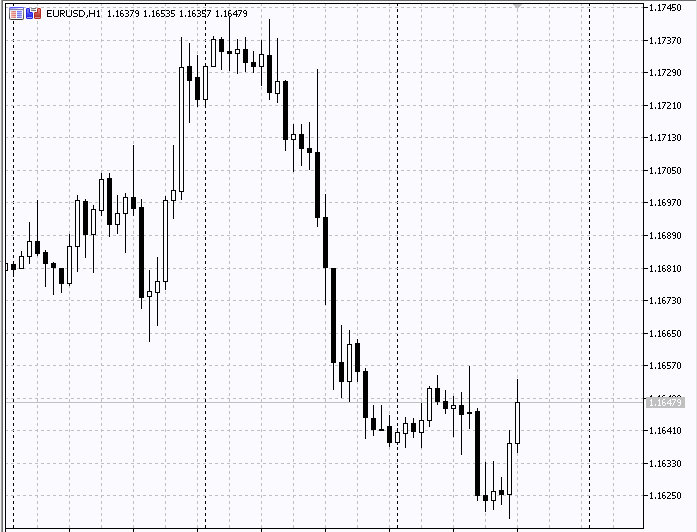### Conclusion

In this article, we offered quite an interesting tool. It allows traders to quickly and easily visualize the presence of a correlation between almost any number of trading instruments. The main purpose of the tool lies in the analysis of trading instruments and in the development of various arbitrage strategies. Of course, this tool alone is insufficient for developing a full-fledged trading system, but it can greatly facilitate the first stage of developing a trading system – the search for correlated instruments and their dependencies.

### Programs used in the article

#
Name
Type
Description
1  PlotBase.mqh  Class library  Base class for building graphs
2  Scatter.mqh  Class library  Class for building scatter plots
3  Histogram.mqh  Class library  Class for building histograms
4  PairPlot.mqh  Class library  PairPlot tool class
5  PairPlotDemo.mqh  Class library  Class for demonstrating the tool connection
6  PairPlot.mq5  Indicator  Indicator demonstrating the work of the tool

Translated from Russian by MetaQuotes Software Corp.
Original article: https://www.mql5.com/ru/articles/4820

Attached files |
MQL5.zip (377.1 KB)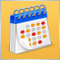950 websites broadcast the Economic Calendar from MetaQuotes

The widget provides websites with a detailed release schedule of 500 indicators and indices, of the world's largest economies. Thus, traders quickly receive up-to-date information on all important events with explanations and graphs in addition to the main website content.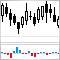Developing stock indicators featuring volume control through the example of the delta indicator

The article deals with the algorithm of developing stock indicators based on real volumes using the CopyTicks() and CopyTicksRange() functions. Some subtle aspects of developing such indicators, as well as their operation in real time and in the strategy tester are also described.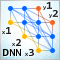Deep Neural Networks (Part VIII). Increasing the classification quality of bagging ensembles

The article considers three methods which can be used to increase the classification quality of bagging ensembles, and their efficiency is estimated. The effects of optimization of the ELM neural network hyperparameters and postprocessing parameters are evaluated.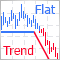Combining trend and flat strategies

There are numerous trading strategies out there. Some of them look for a trend, while others define ranges of price fluctuations to trade within them. Is it possible to combine these two approaches to increase profitability?